Courses

# NEET Previous Year Questions: Centre of Mass

## 12 Questions MCQ Test Physics For JEE | NEET Previous Year Questions: Centre of Mass

Description
This mock test of NEET Previous Year Questions: Centre of Mass for JEE helps you for every JEE entrance exam. This contains 12 Multiple Choice Questions for JEE NEET Previous Year Questions: Centre of Mass (mcq) to study with solutions a complete question bank. The solved questions answers in this NEET Previous Year Questions: Centre of Mass quiz give you a good mix of easy questions and tough questions. JEE students definitely take this NEET Previous Year Questions: Centre of Mass exercise for a better result in the exam. You can find other NEET Previous Year Questions: Centre of Mass extra questions, long questions & short questions for JEE on EduRev as well by searching above.
QUESTION: 1

### Two identical particles move towards each other with velocity 2v and vrespectively. The velocity of centre of mass is  [AIEEE 2002]

Solution:

Let say that direction of any one particle to be positive, let say right. Thus the equation of velocity for the center of mass = m x 2v + m x (-v) / m + m
= mv / 2m = v/2

QUESTION: 2

### Consider the following two statements : A. Linear momentum of a system of particles is zero. B. kinetic energy of a system of particles is zero.Then  [AIEEE 2003]

Solution:

We know momentum of a system = mass * change in velocity
And Kinetic Energy = 1/2 * mass * (velocity)²
If change in velocity is zero that doesn't mean that the velocity of the system is zero. If the velocity of a system is zero then there is no change in velocity.

QUESTION: 3

### A body A of mass M while falling vertically downwards under gravity breaks into two parts; a body B of mass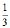M and, a body C of mass M. The centre of mass of bodies B and C taken together shifts compared to that of body A towards   [AIEEE 2005]

Solution:

Since there is no different external force applied to the individual particles, the centre of mass remains same and does not shift.

QUESTION: 4

A mass m moves with a velocity v and collides inelastically with another identical mass. After collision the 1st mass moves with velocity in a direction perpendicular to the initial direction of motion. Find the speed of the second mass after collision.

[AIEEE 2005]

Solution: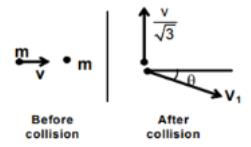0=mv/√3−mv1sinθ
V1=2v/√3

QUESTION: 5

Consider a two particle system with particles having masses m1 and m2. If the first particle is pushed towards the centre of mass through a distance d, by what distance should the second particle be moved, so as to keep the centre of mass at the same position?

[AIEEE 2006]

Solution: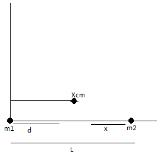Xcm = (m1x1 + m2x2)/(m1 + m2)
Xcm = m2L/(m1 + m2)
Now, ATQ
Xcm = (m1d + m2(L-x))/(m1 + m2)
Since Xcm is same so,
⇒ (m1d + m2(L-x))/(m1 + m2) = m2L/(m1 + m2)
⇒ m1d + m2L - m2x = m2L
⇒ m1d = m2x
⇒ x = m1d/m2

QUESTION: 6

A bomb of mass 16 kg at rest explodes into two pieces of masses 4 kg and 12 kg. The velocity of the 12 kg mass is 4 ms-1. The kinetic energy of the other mass is

[AIEEE 2006]

Solution:

Applying Conservation of Linear Momentum
16 x 0 = 12 x 4 + 4 x v => v = -12 m/s
So, KE = ½ m v2 = ½ x 4 x 144 = 288J

QUESTION: 7

A player caught a cricket ball of mass 150 g moving at a rate of 20 m/s. If the catching process is completed in 0.1 s, the force of the blow exerted by the ball on the hand of the player is equal to

[AIEEE 2006]

Solution:

F = ma = m(v/t)
F = 150 × 20 / 0.1 × 1000
F = 30N

QUESTION: 8

A circular disc of radius R is removed from a bigger circular disc of radius 2 R, such that the circumference of the discs coincide. The centre of mass of the new disc is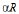from the centre of the bigger disc. The value of a is

[AIEEE 2007]

Solution: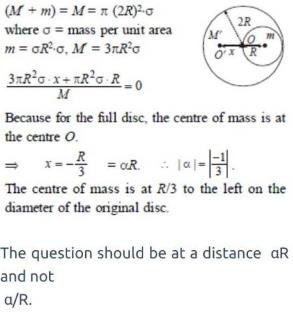QUESTION: 9

A thin rod of length L is lying along the x-axis with its ends at x = 0 and x = L. Its linear density (mass/length) varies with x as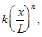where n can be zero or any positive number. If the position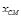of the centre of mass of the rod is plotted against n, which of the following graphs best approximates the dependence ofon n?

[AIEEE 2008]

Solution:
QUESTION: 10

A block of mass 0.50 kg is moving with a speed of 2.00 ms-1 on a smooth surface. It strikes another mass of 1.00 kg and then they move together as a single body. The energy loss during the collision is

[AIEEE 2008]

Solution:
QUESTION: 11

Statement I : Two particles moving in the same direction do not lose all their energy in a completely inelastic collision.

Statement II : Principle of conservation of momentum holds true for all kinds of collisions.

[AIEEE 2010]

Solution:

The strong interaction force between two bodies in which momentum is conserved is called collision. In any type of collision momentum is always conserved kinetic energy may or may not be conserved.

QUESTION: 12

Statement-I : A point particle of mass m moving with speed u collides with stationary point particle of mass M. If the maximum energy loss possible is given as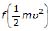then f =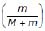.

Statement-II : Maximum energy loss occurs when the particles get stuck together as a result of the collision.                                      [JEE MAIN 2013]

Solution: